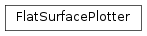# mvpa2.misc.plot.flat_surf.FlatSurfacePlotter¶class `mvpa2.misc.plot.flat_surf.``FlatSurfacePlotter`(surface, curvature=None, min_nsteps=500, range_='2_98%', threshold=None, color_map=None, max_deformation=0.5)

Plots data on a flat surface

Methods

`__call__`(data)
`set_underlay`(u) Sets the underlay
Parameters: surface: surf.Surface a flat surface curvature: str or np.ndarray (Filename of) data representing curvature at each node. min_steps: int Minimal side of output plots in pixel range_: str or float or tuple If a tuple (a,b), then this tuple is returned. If a float a, then (-a,a) is returned. “R(a)”, where R(a) denotes the string representation of float a, is equivalent to range_=a. “R(a)_R(b)” is equivalent to range_=(a,b). “R(a)_R(b)%” indicates that the a-th and b-th percentile of xs is taken to define the range. “R(a)%” is equivalent to “R(a)_R(100-a)%” threshold: str or float or tuple Indicates which values will be shown. Syntax as in range_ color_map: str colormap to use max_deformation: float maximum deformation to make a non-flat surface flat. The normals of each face must have a dot product with the average face normal that is not less than (1-max_deformation); otherwise an exception is raised. The rationale for this option is that certain surfaces may be almost flat, and projecting the vertices on a truly flat surface should be fine. On the other hand, surfaces that are definitly not flat (such as full cortical surface models) should cause an error to be raised when it is attempted to flatten them

Methods

`__call__`(data)
`set_underlay`(u) Sets the underlay
`set_underlay`(u)

Sets the underlay